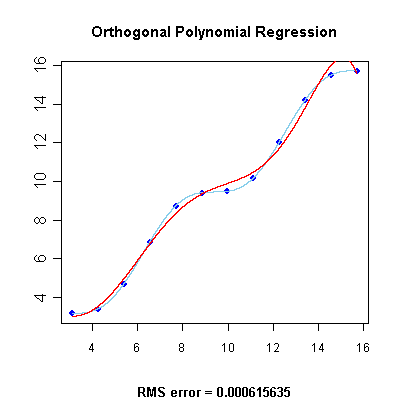•http://facebook.com/
•https://www.google.com/accounts/o8/id
•https://me.yahoo.comCOST (GBP)4.760.000

# Orthogonal

viewed 3268 times and licensed 80 times
Approximates an arbitrary function using orthogonal polynomials.
Controller: CodeCogsC++

## Class Orthogonal

This class approximates an arbitrary discrete function by least squares fitting orthogonal polynomials. In mathematics, two polynomials f and g are orthogonal to each other with respect to a nonnegative <em> weight function </em> w precisely if

In other words, if polynomials are treated as vectors and the inner product of two polynomials and is defined as

then the orthogonal polynomials are simply orthogonal vectors in this inner product space. The algorithm uses this class of polynomials to achieve the best curve fitting by minimization in terms of least squares.

Below you will find the regression graph for a set of points obtained by evaluating the function , displayed in light blue, at particular abscissas. The regression polynomial, displayed in red, has been calculated using this class. The root mean squared error is also displayed.As a general rule, the degree parameter should always be strictly less than the the total number of sample points.

## References:

• Tuan Dang Trong, "Numath Library" in Fortran 77

### Example 1

The following example displays 10 approximated values (you may change this amount through the N_out variable) for the given function with abscissas equally spaced in the interval. The absolute approximation errors are also displayed. The X and Y coordinate arrays are initialized by evaluating this function for N = 13 points equally spaced in the domain from to . The degree parameter (the number of orthogonal polynomials to use) is equal to 12 in this example.

#include <codecogs/maths/regression/orthogonal.h>

#include "orthogonal.h"

#include <cmath>
#include <iostream>
#include <iomanip>
using namespace std;

#define PI 3.1415926535897932384626433832795
#define N  13

int main()
{
// Declare and initialize two arrays to hold the coordinates of the initial data points
double x[N], y[N];

double xx = PI, step = 4 * PI / (N - 1);
for (int i = 0; i < N; i++, xx += step) {
x[i] = xx;
y[i] = sin(xx) + xx;
}

// Initialize the regression approximation routine with known data points
Maths::Regression::Orthogonal A(N, x, y, N - 1);

// Interrogate the regression function to find approximated values
int N_out = 10;
xx = PI, step = (3 * PI) / (N_out - 1);
for (int i = 0; i < N_out; ++i, xx += step) {
cout << "x = " << setw(7) << xx << "  y = ";
cout << setw(7) << A.getValue(xx);
cout << setw(10) << "error = " << fabs(sin(xx) + xx - A.getValue(xx)) << endl;
}

return 0;
}
Output:
x = 3.14159  y = 3.14159  error = 1.33227e-15
x = 4.18879  y = 3.32276  error = 1.77636e-15
x = 5.23599  y = 4.36996  error = 1.86517e-14
x = 6.28319  y = 6.28319  error = 7.19425e-14
x = 7.33038  y = 8.19641  error = 1.1191e-13
x = 8.37758  y = 9.24361  error = 2.41585e-13
x = 9.42478  y = 9.42478  error = 2.91323e-13
x =  10.472  y = 9.60595  error = 2.62901e-13
x = 11.5192  y = 10.6531  error = 2.2915e-13
x = 12.5664  y = 12.5664  error = 1.04805e-13

### Authors

Lucian Bentea (August 2005)
##### Source Code

Source code is available when you agree to a GP Licence or buy a Commercial Licence.

Not a member, then Register with CodeCogs. Already a Member, then Login.

## Members of Orthogonal

#### Orthogonal

 Orthogonal( int n double* x double* y int degree )[constructor]
Initializes the necessary data for following evaluations of the polynomial.
 n The number of initial points in the arrays x and y x The x-coordinates for the initial points y The y-coordinates for the initial points degree The number of orthogonal polynomials to use in the approximation (must be strictly less than the number of points n)

#### GetValue

 doublegetValue( double x )
Returns the approximated ordinate at the given abscissa.
 x The abscissa of the approximation point

## Orthogonal Once

 doubleOrthogonal_once( int n double* x double* y int degree double a )
This function implements the Orthogonal class for one off calculations, thereby avoid the need to instantiate the Orthogonal class yourself.

### Example 2

The following graphs are constructed from the interpolation of the following values, using a 3rd order polynomial and then 8th order polynomial, respectively:
x = 1  y = 0.22
x = 2  y = 0.04
x = 3  y = -0.13
x = 4  y = -0.17
x = 5  y = -0.04
x = 6  y = 0.09
x = 7  y = 0.11
There is an error with your graph parameters for Orthogonal_once with options n=7 x="1 2 3 4 5 6 7" y="0.22 0.04 -0.13 -0.17 -0.04 0.09 0.11" degree=3 a=1:7 .input

Error Message:Function Orthogonal_once failed. Ensure that: Invalid C++

There is an error with your graph parameters for Orthogonal_once with options n=7 x="1 2 3 4 5 6 7" y="0.22 0.04 -0.13 -0.17 -0.04 0.09 0.11" degree=6 a=1:7 .input

Error Message:Function Orthogonal_once failed. Ensure that: Invalid C++

### Parameters

 n The number of initial points in the arrays x and y x The x-coordinates for the initial points y The y-coordinates for the initial points degree The number of polynomials to use in the approximation (must be strictly less than the number of points n) a The x-coordinate for the output point

### Returns

the interpolated y-coordinate that corresponds to a.
##### Source Code

Source code is available when you agree to a GP Licence or buy a Commercial Licence.

Not a member, then Register with CodeCogs. Already a Member, then Login.

Last Modified: 23 Jan 10 @ 14:33     Page Rendered: 2022-03-14 14:57:38